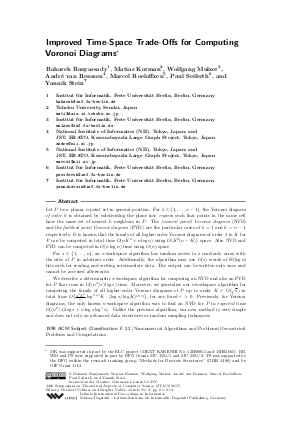Document# Improved Time-Space Trade-Offs for Computing Voronoi Diagrams

### Authors Bahareh Banyassady, Matias Korman, Wolfgang Mulzer, André van Renssen, Marcel Roeloffzen, Paul Seiferth, Yannik Stein## File

LIPIcs.STACS.2017.9.pdf
• Filesize: 0.57 MB
• 14 pages

## Cite As

Bahareh Banyassady, Matias Korman, Wolfgang Mulzer, André van Renssen, Marcel Roeloffzen, Paul Seiferth, and Yannik Stein. Improved Time-Space Trade-Offs for Computing Voronoi Diagrams. In 34th Symposium on Theoretical Aspects of Computer Science (STACS 2017). Leibniz International Proceedings in Informatics (LIPIcs), Volume 66, pp. 9:1-9:14, Schloss Dagstuhl - Leibniz-Zentrum für Informatik (2017)
https://doi.org/10.4230/LIPIcs.STACS.2017.9

## Abstract

Let P be a planar n-point set in general position. For k between 1 and n-1, the Voronoi diagram of order k is obtained by subdividing the plane into regions such that points in the same cell have the same set of nearest k neighbors in P. The (nearest point) Voronoi diagram (NVD) and the farthest point Voronoi diagram (FVD) are the particular cases of k=1 and k=n-1, respectively. It is known that the family of all higher-order Voronoi diagrams of order 1 to K for P can be computed in total time O(n K^2 + n log n) using O(K^2(n-K)) space. Also NVD and FVD can be computed in O(n log n) time using O(n) space. For s in {1, ..., n}, an s-workspace algorithm has random access to a read-only array with the sites of P in arbitrary order. Additionally, the algorithm may use O(s) words of Theta(log n) bits each for reading and writing intermediate data. The output can be written only once and cannot be accessed afterwards. We describe a deterministic s-workspace algorithm for computing an NVD and also an FVD for P that runs in O((n^2/s) log s) time. Moreover, we generalize our s-workspace algorithm for computing the family of all higher-order Voronoi diagrams of P up to order K in O(sqrt(s)) in total time O( (n^2 K^6 / s) log^(1+epsilon)(K) (log s / log K)^(O(1)) ) for any fixed epsilon > 0. Previously, for Voronoi diagrams, the only known s-workspace algorithm was to find an NVD for P in expected time O((n^2/s) log s + n log s log^*s). Unlike the previous algorithm, our new method is very simple and does not rely on advanced data structures or random sampling techniques.
##### Keywords
• memory-constrained model
• Voronoi diagram

## Metrics

• Access Statistics
• Total Accesses (updated on a weekly basis)
0

## References

1. Alok Aggarwal, Leonidas J. Guibas, James B. Saxe, and Peter W. Shor. A linear-time algorithm for computing the voronoi diagram of a convex polygon. Discrete Comput. Geom., 4:591-604, 1989.2. Boris Aronov, Matias Korman, Simon Pratt, André van Renssen, and Marcel Roeloffzen. Time-space trade-offs for triangulating a simple polygon. In Proc. 15th Scand. Sympos. Workshops Algorithm Theory (SWAT), volume 53, pages 30:1-30:12, 2016.3. Sanjeev Arora and Boaz Barak. Computational Complexity.A modern approach. Cambridge University Press, 2009.4. Tetsuo Asano, Kevin Buchin, Maike Buchin, Matias Korman, Wolfgang Mulzer, Günter Rote, and André Schulz. Memory-constrained algorithms for simple polygons. Comput. Geom., 46(8):959-969, 2013.5. Tetsuo Asano and David Kirkpatrick. Time-space tradeoffs for all-nearest-larger-neighbors problems. In Proc. 13th Int. Symp. Algorithms and Data Structures (WADS), pages 61-72, 2013.6. Tetsuo Asano, Wolfgang Mulzer, Günter Rote, and Yajun Wang. Constant-work-space algorithms for geometric problems. J. of Comput. Geom., 2(1):46-68, 2011.7. Tetsuo Asano, Wolfgang Mulzer, and Yajun Wang. Constant-work-space algorithms for shortest paths in trees and simple polygons. J. Graph Algorithms Appl., 15(5):569-586, 2011.8. Franz Aurenhammer, Rolf Klein, and Der-Tsai Lee. Voronoi diagrams and Delaunay triangulations. World Scientific Publishing Co. Pte. Ltd., Hackensack, NJ, 2013.9. Yeganeh Bahoo, Bahareh Banyassady, Prosenjit Bose, Stephane Durocher, and Wolfgang Mulzer. Finding the k-visibility region of a point in a simple polygon in the memory-constrained model. In Proc. 32nd European Workshop Comput. Geom. (EWCG), 2016.10. Luis Barba, Matias Korman, Stefan Langerman, Kunihiko Sadakane, and Rodrigo I. Silveira. Space-time trade-offs for stack-based algorithms. Algorithmica, 72(4):1097-1129, 2015.11. Luis Barba, Matias Korman, Stefan Langerman, and Rodrigo I. Silveira. Computing the visibility polygon using few variables. Comput. Geom., 47(9):918-926, 2013.12. Mark de Berg, Otfried Cheong, Marc van Kreveld, and Mark Overmars. Computational geometry. Algorithms and applications. Springer-Verlag, third edition, 2008.13. Allan Borodin and Stephen A. Cook. A time-space tradeoff for sorting on a general sequential model of computation. SIAM J. Comput., 11:287-297, 1982.14. Hervé Brönnimann, Timothy M. Chan, and Eric Y. Chen. Towards in-place geometric algorithms and data structures. In Proc. 20th Annu. Sympos. Comput. Geom. (SoCG), pages 239-246, 2004.15. Timothy M Chan. Remarks on k-level algorithms in the plane. manuscript, Univ. of Waterloo, 1999.16. Timothy M Chan. Random sampling, halfspace range reporting, and construction of (≤ k)-levels in three dimensions. SIAM J. Comput., 30(2):561-575, 2000.17. Timothy M. Chan and Eric Y. Chen. Multi-pass geometric algorithms. Discrete Comput. Geom., 37(1):79-102, 2007.18. Omar Darwish and Amr Elmasry. Optimal time-space tradeoff for the 2D convex-hull problem. In Proc. 22nd Annu. European Sympos. Algorithms (ESA), pages 284-295, 2014.19. Amr Elmasry and Frank Kammer. Space-efficient plane-sweep algorithms. arXiv : 1507.01767, 2015.20. Sariel Har-Peled. Shortest path in a polygon using sublinear space. J. of Comput. Geom., 7(2):19-45, 2016.21. Matias Korman. Memory-constrained algorithms. In Encyclopedia of Algorithms, pages 1260-1264. Springer Berlin Heidelberg, 2016. URL: http://dx.doi.org/10.1007/978-3-642-27848-8_586-1.
22. Matias Korman, Wolfgang Mulzer, André van Renssen, Marcel Roeloffzen, Paul Seiferth, and Yannik Stein. Time-space trade-offs for triangulations and Voronoi diagrams. In Proc. 14th Int. Symp. Algorithms and Data Structures (WADS), pages 482-494, 2015.23. Der-Tsai Lee. On k-nearest neighbor Voronoi diagrams in the plane. IEEE Trans. Computers, 31(6):478-487, 1982.24. J. Ian Munro and Mike Paterson. Selection and sorting with limited storage. Theoret. Comput. Sci., 12:315-323, 1980.25. J. Ian Munro and Venkatesh Raman. Selection from read-only memory and sorting with minimum data movement. Theoret. Comput. Sci., 165(2):311-323, 1996.26. J. Pagter and T. Rauhe. Optimal time-space trade-offs for sorting. In Proc. 39th Annu. IEEE Sympos. Found. Comput. Sci. (FOCS), pages 264-268, 1998.27. Ira Pohl. A minimum storage algorithm for computing the median. Technical Report RC2701, IBM, 1969.28. John E. Savage. Models of computation - exploring the power of computing. Addison-Wesley, 1998.X

Feedback for Dagstuhl Publishing